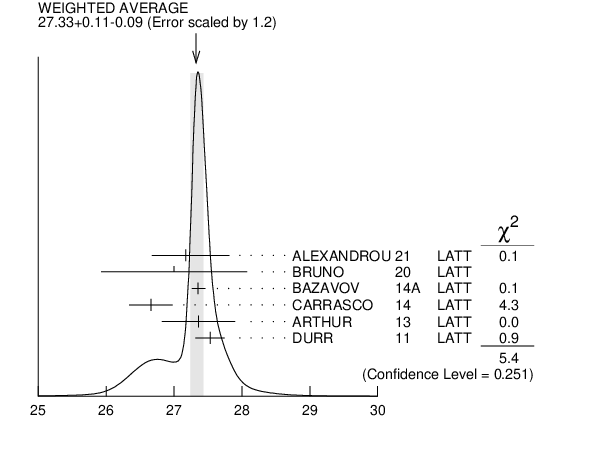# ${\boldsymbol m}_{{{\boldsymbol s}}}/{{\overline{\boldsymbol m}}}$ MASS RATIO INSPIRE search

${{\overline{\mathit m}}}{}\equiv$ (${\mathit m}_{{{\mathit u}}}$ $+$ ${\mathit m}_{{{\mathit d}}})/$2
VALUE DOCUMENT ID TECN
$\bf{ 27.3 {}^{+0.7}_{-1.3}}$ OUR EVALUATION
$27.35$ $\pm0.05$ ${}^{+0.10}_{-0.07}$ 1
 2014 A
LATT
$26.66$ $\pm0.32$ 2
 2014
LATT
$27.36$ $\pm0.54$ 3
 2013
LATT
$27.53$ $\pm0.20$ $\pm0.08$ 4
 2011
LATT
• • • We do not use the following data for averages, fits, limits, etc. • • •
$26.8$ $\pm1.4$ 5
 2011 A
LATT
$27.3$ $\pm0.9$ 6
 2010
LATT
$28.8$ $\pm1.65$ 7
 2008
LATT
$27.3$ $\pm0.3$ $\pm1.2$ 8
 2008
LATT
$23.5$ $\pm1.5$ 9
 2007 A
THEO
$27.4$ $\pm0.4$ 10
 2004
LATT
1  BAZAVOV 2014A is a lattice computation using 4 dynamical flavors of HISQ fermions.
2  CARRASCO 2014 is a lattice QCD computation of light quark masses using 2 + 1 + 1 dynamical quarks, with ${{\mathit m}_{{u}}}$ = ${{\mathit m}_{{d}}}{}\not=$ ${{\mathit m}_{{s}}}{}\not=$ ${{\mathit m}_{{c}}}$. The ${\mathit {\mathit u}}$ and ${\mathit {\mathit d}}$ quark masses are obtained separately by using the ${{\mathit K}}$ meson mass splittings and lattice results for the electromagnetic contributions.
3  ARTHUR 2013 is a lattice computation using 2+1 dynamical domain wall fermions.
4  DURR 2011 determine quark mass from a lattice computation of the meson spectrum using ${{\mathit N}_{{f}}}$ = 2 + 1 dynamical flavors. The lattice simulations were done at the physical quark mass, so that extrapolation in the quark mass was not needed.
5  AOKI 2011A determine quark masses from a lattice computation of the hadron spectrum using ${{\mathit N}_{{f}}}$ = 2 + 1 dynamical flavors of domain wall fermions.
6  BLOSSIER 2010 determines quark masses from a computation of the hadron spectrum using ${{\mathit N}_{{f}}}$=2 dynamical twisted-mass Wilson fermions.
7  ALLTON 2008 use a lattice computation of the ${{\mathit \pi}}$, ${{\mathit K}}$, and ${{\mathit \Omega}}$ masses with 2+1 dynamical flavors of domain wall quarks, and non-perturbative renormalization.
8  BLOSSIER 2008 use a lattice computation of pseudoscalar meson masses and decay constants with 2 dynamical flavors and non-perturbative renormalization.
9  OLLER 2007A use unitarized chiral perturbation theory to order $\mathit p{}^{4}$.
10  Three flavor dynamical lattice calculation of pseudoscalar meson masses.${\mathit m}_{{{\mathit s}}}/{{\overline{\mathit m}}}$ MASS RATIO
References:
 BAZAVOV 2014A
PR D90 074509 Charmed and Light Pseudoscalar Meson Decay Constants from Four-flavor Lattice QCD with Physical Light Quarks
 CARRASCO 2014
NP B887 19 Up, Down, Strange and Charm Quark Masses with $\mathit N_{f}$ = 2+1+1 Twisted Mass Lattice QCD
 ARTHUR 2013
PR D87 094514 Domain Wall QCD with Near-Physical Pions
 AOKI 2011A
PR D83 074508 Continuum Limit Physics from 2+1 Flavor Domain Wall QCD
 DURR 2011
PL B701 265 Lattice QCD at the Physical Point: Light Quark Masses
 BLOSSIER 2010
PR D82 114513 Average up/down, strange, and charm Quark Masses with $\mathit N_{f}$=2 Twisted-Mass Lattice QCD
 ALLTON 2008
PR D78 114509 Physical Results from 2+1 Flavor Domain Wall QCD and SU(2) Chiral Perturbation Theory
 BLOSSIER 2008
JHEP 0804 020 Light Quark Masses and Pseudoscalar Decay Constants from $\mathit N_{f}$ = 2 Lattice QCD with Twisted Mass Fermions
 OLLER 2007A
EPJ A34 371 Non-Perturbative Study of the Light Pseudoscalar Masses in Chiral Dynamics
 AUBIN 2004
PR D70 031504 First Determination of the Strange and Light Quark Masses from Full Lattice QCD